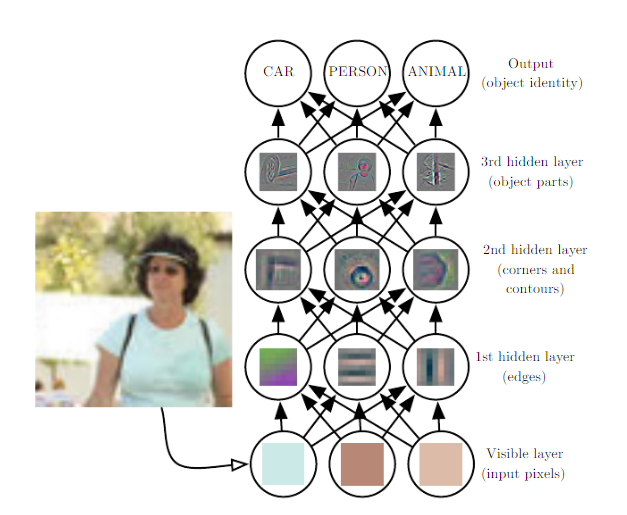TensorFlow.JS

TensorFlow is an open-source javaScript library for machine learning based on an original library developed by Google in C++ with bindings in Python.
"tensorflow.JS" is a javascript library capable of learning in the browser with training sets and test sets.

What are tensors?->In mathematics, tensors are geometric elements (numbers, vectors, matrices, etc.). These tensors are not used by the CPU but the GPU (Graphics Processing Unit). GPU make use of the WEBGL library based in OPENGL Technology. Images are sets of numbers in matrices, every single pixel correspond to an RGBA vector. With the training sets we have thousands of sample images that are used for training our neural network. Every hidden layer of the neural network keeps track of a different feature (e.g; contour, corners, groups of pixels, blobs, etc.).After training the tensors are been optimised with a function with a function producing the minimum distance between all the images like in a linear regression or a polynomial regression. As a student, I'll try to explain an example using neural network e.g.:
• MNIST example uses sixty thousand samples of handwritten numbers from zero to nine.
•• This is an example of computer vision software developed from our school capable of analyzing high performance thin layer chromatography, that is to analyze the composition of a plant automatically from HPTLC Algorithm
• This is an example of machine learning and computer vision that helps classify and recognize vegetal and animal species